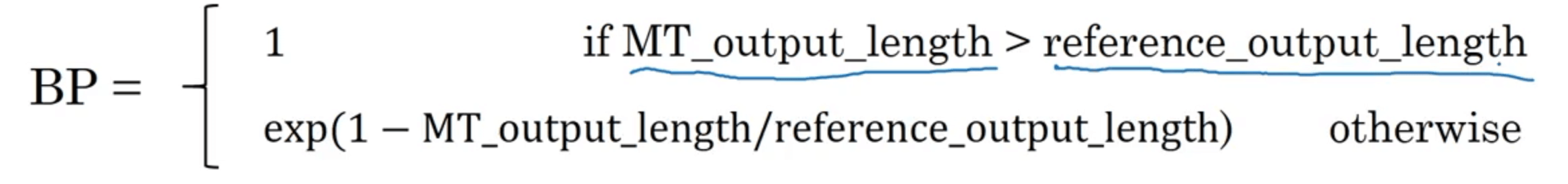# 【深度学习基础】第四十七课：BLEU得分

## BLEU得分

Posted by x-jeff on March 3, 2021

【深度学习基础】系列博客为学习Coursera上吴恩达深度学习课程所做的课程笔记。

# 1.BLEU得分

BLEU原文：Papineni K, Roukos S, Ward T, et al. Bleu: a method for automatic evaluation of machine translation[C]//Proceedings of the 40th annual meeting of the Association for Computational Linguistics. 2002: 311-318.

• French : Le chat est sur le tapis.
• Reference 1 : The cat is on the mat.
• Reference 2 : There is a cat on the mat.

BLEU : Bilingual Evaluation Understudy。

unigram count count_clip
the 7 2

“unigram”表示我们每次只分析一个单词，”count”为该单词在机器翻译结果中出现的次数，”count_clip”为该单词在Reference中出现的最高频次。优化后的精确度为：$\frac{2}{7}$。

bigram count count_clip
the cat 2 1
cat the 1 0
cat on 1 1
on the 1 1
the mat 1 1

$\frac{1+0+1+1+1}{2+1+1+1+1}=\frac{4}{6}=\frac{2}{3}$

$P_n=\frac{\sum _{n-gram \in \hat y} count\_clip \ (n-gram) }{ \sum _{n-gram \in \hat y} count \ (n-gram) }$

$BLEU \ score=BP \cdot exp(\frac{1}{k}\sum^k_{n=1} P_n)$

BP（Brevity Penalty，简短惩罚）是一个惩罚因子。添加BP惩罚因子的原因在于如果模型输出了一个非常短的翻译，那么它会更容易得到一个高精确度。因为输出的大部分词可能都出现在Reference中。因此我们需要BP惩罚因子对非常短的翻译结果进行惩罚。BP惩罚因子的定义如下：BLEU得分对于机器翻译任务来说，是一个相当不错的单一实数评估指标。此外，BLEU得分也经常用于图像描述系统。